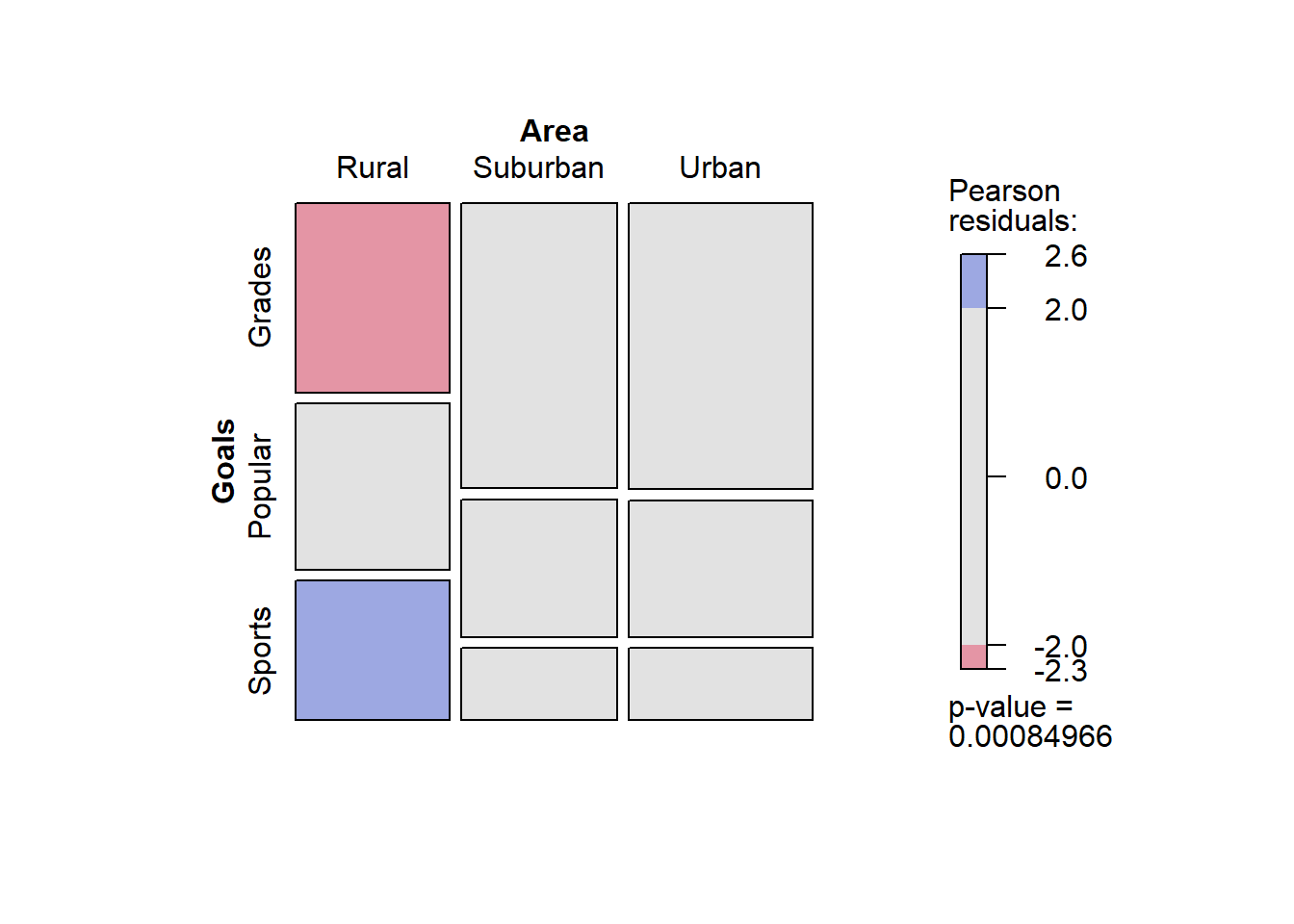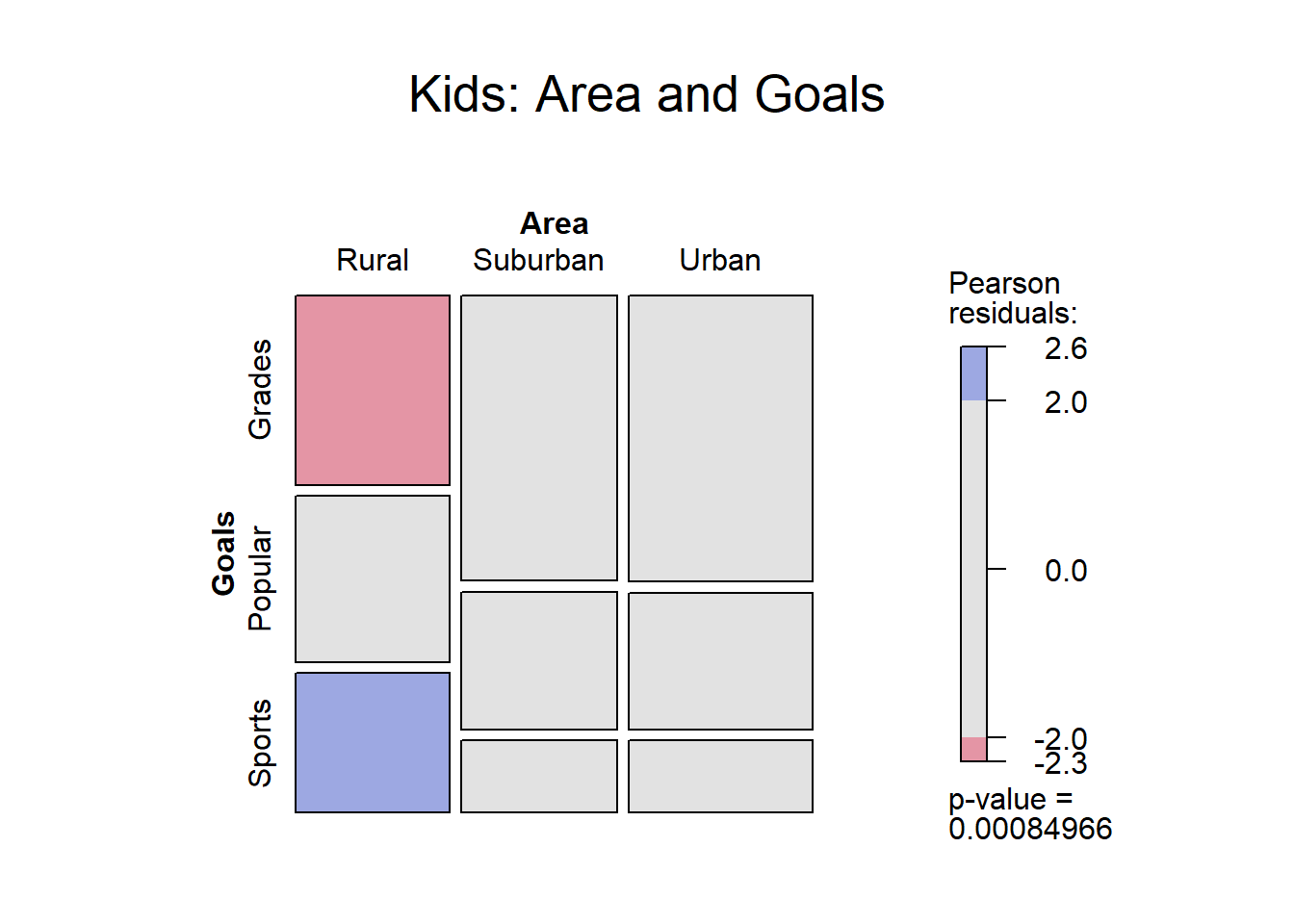# ADA1: Class 25, Two-way categorical tables

Advanced Data Analysis 1, Stat 427/527, Fall 2022, Prof. Erik Erhardt, UNM

Author

Published

August 13, 2022

Include your answers in this document in the sections below the rubric where I have point values indicated (1 p).

# Rubric

Answer the questions with the data example.

# Goals vs School area (rural, suburban, urban)

Repeat the analysis above, but compare School area instead of Grade level.

# Tabulate by two categorical variables:
tab_GoalsArea <-
xtabs(
~ Goals + Area
, data = dat_kids
)
tab_GoalsArea
         Area
Goals     Rural Suburban Urban
Popular    50       42    49
Sports     42       22    26
# column proportions
prop.table(
tab_GoalsArea
, margin = 2
) %>%
signif(2)
         Area
Goals     Rural Suburban Urban
Popular  0.34     0.28  0.28
Sports   0.28     0.15  0.15
1. (1 p) Set up the null and alternative hypotheses in words and notation.

• In words: There is an association between [row variable] and [column variable].’’
• In notation: $$H_0: p(i \textrm{ and } j) = p(i)p(j)$$ versus $$H_A: p(i \textrm{ and } j) \ne p(i)p(j)$$, for all row categories $$i$$ and column categories $$j$$.
2. Choose the significance level of the test, such as $$\alpha=0.05$$.

3. Compute the test statistic, such as $$X^2$$.

chisq_ga <-
chisq.test(
tab_GoalsArea
, correct = FALSE
)
chisq_ga

Pearson's Chi-squared test

data:  tab_GoalsArea
X-squared = 18.828, df = 4, p-value = 0.0008497
  # names(chisq_ga) for the objects to report

The test statistic is $$X^2 = 18.8$$.

1. The p-value $$= 8.5\times 10^{-4}$$.

2. (1 p) State the conclusion in terms of the problem.

3. Check assumptions of the test (expected count for each cell is at least 5, or at least 1 provided the expected counts are not too variable).

# table of expected frequencies:
chisq_ga$expected  Area Goals Rural Suburban Urban Grades 76.99372 78.02720 91.97908 Popular 43.95188 44.54184 52.50628 Sports 28.05439 28.43096 33.51464 # smallest expected frequency: min(chisq_ga$expected)
 28.05439

(1 p) Are the model assumptions met?

## Deviations details

If you rejected the null hypothesis above, the Pearson residuals are a way to indicate which cells of the table were different from expected. Residuals more extreme than roughly $$\pm 2$$ are considered large’’.

# The Pearson residuals
chisq_ga$residuals %>% signif(3)  Area Goals Rural Suburban Urban Grades -2.280 1.020 1.150 Popular 0.912 -0.381 -0.484 Sports 2.630 -1.210 -1.300 # The sum of the squared residuals is the chi-squared statistic: chisq_ga$residuals^2 %>% signif(3)
         Area
Goals     Rural Suburban Urban
Popular 0.832    0.145 0.234
Sports  6.930    1.450 1.680
sum(chisq_ga\$residuals^2)
 18.82763

(1 p) Interpret the Pearson residuals.

The mosaic plot is a visual representation of the observed frequencies (areas of each box) and the Pearson residual (color bar). If rectangles are the same size along rows and columns (and gray), then they’re close to expected. Differences between observed and expected frequencies are indicated by different sized rectangles across rows or down columns and colors indicate substantial contributions to the $$X^2$$ statistic.

# mosaic plot
library(vcd)

# this layout gives us the interpretation we want:
mosaic(~ Area + Goals, data = dat_kids, shade=TRUE, legend=TRUE, direction = "v")vcd::mosaic(
~ Area + Goals
, data      = dat_kids
, main      = "Kids: Area and Goals"
)